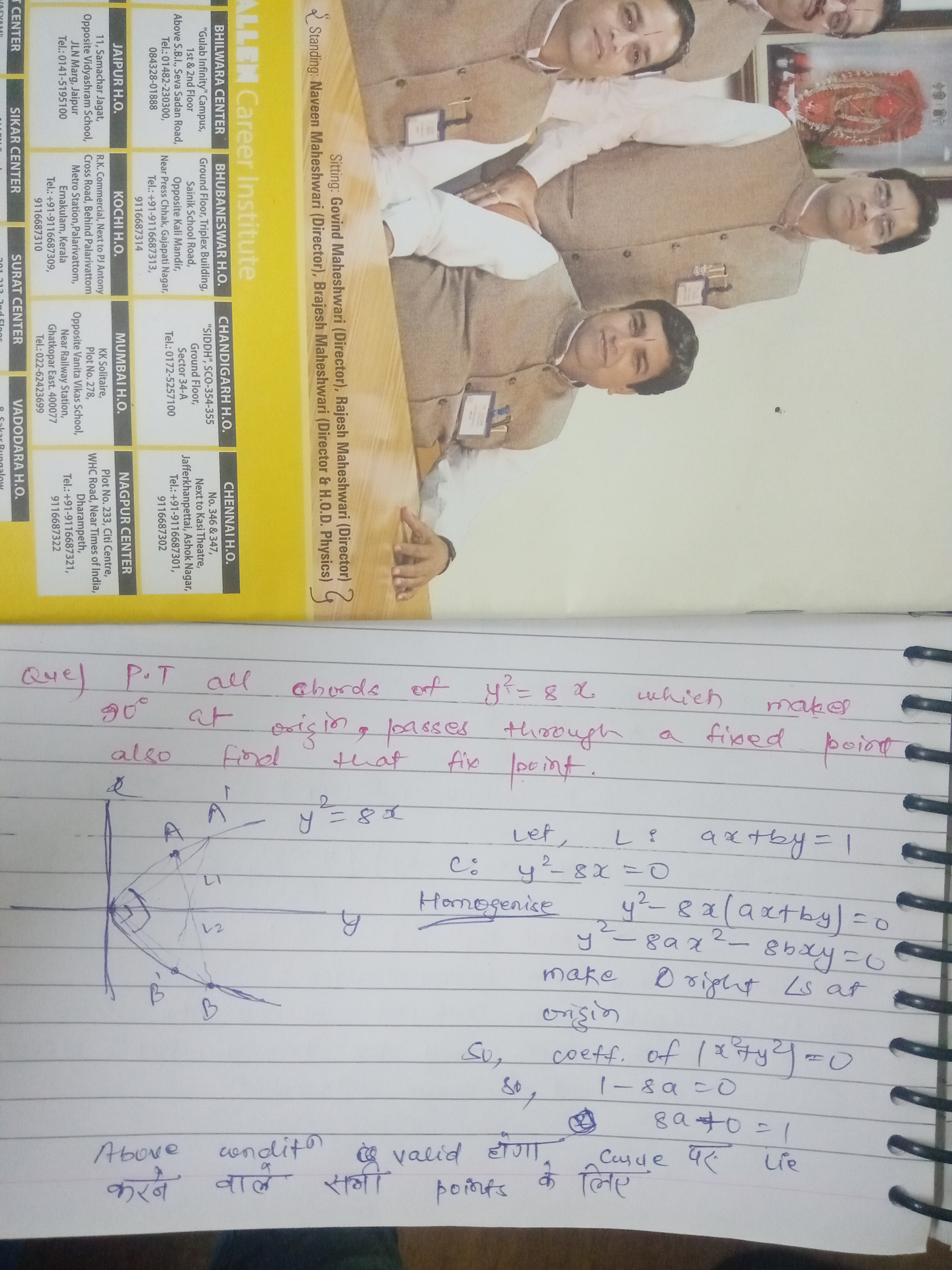# Doubt from Straight lineDo you know the concept of homogenisation?!
If yes then basically you have to assume a like ax+by=1 and find the combined equation of the lines from origin to the point where this line cuts the curve by homogenisation.
Now from the concept of pair of straight line , if these lines subtends 90 degree at the origin then coeff of x² + coeff of y² = 0
And hence we get a=1/8

Therefore the variable lines must be 1/8 x + by =1 where b is variable
This line always passes through a fixed point i.e (8,0)

Thanks